# Fourier series

In mathematics, a Fourier series is a way to represent a periodic function as the sum of simple sine and cosine functions. It is named after Joseph Fourier, who introduced the concept in the 18th century. The basic idea behind a Fourier series is that any periodic function can be broken down into a sum of sine and cosine functions with different frequencies and amplitudes. The coefficients of these sine and cosine functions can be found using a technique called Fourier analysis, which involves integrating the function over one period and applying trigonometric identities. Once the coefficients are known, the function can be reconstructed using the summation formula of the Fourier series. The Fourier series is widely used in many areas of mathematics, physics, and engineering, such as signal processing and image compression.

Fourier series are used in a wide range of applications in daily life, some of which include:

1. Sound and audio processing: Fourier series are used to analyze and manipulate sound waves, such as in music synthesis and audio compression.

2. Image processing: Fourier transforms can be used to analyze and manipulate images, such as in image compression and computer vision.

3. Data compression: Fourier transforms can be used to compress data by identifying and removing redundant information.

4. Telecommunications: Fourier transforms are used in telecommunications to transmit and receive signals over long distances.

5. Control systems: Fourier series are used to analyze and control systems that exhibit periodic behavior, such as in power systems and mechanical systems.

6. Medical imaging: Fourier transforms are used in medical imaging, such as in Magnetic Resonance Imaging(MRI) and Computed Tomography (CT) scans to provide detailed images of internal organs.

7. Weather forecasting: The Fourier Transform is used in weather forecasting to analyze and predict patterns in weather data, such as temperature and precipitation.

8. Cryptography: The Fourier transform is used in some encryption methods.

These are just a few examples of the many ways in which Fourier series are used in daily life. The versatility and power of this mathematical tool make it a valuable tool in many fields.

Let f(x) be an periodic function interval [ -π, π]. Then Fourier series of f(x) in this interval is given by

f(x) = ao/2 + Σ (an cos nx +bn sin nx) where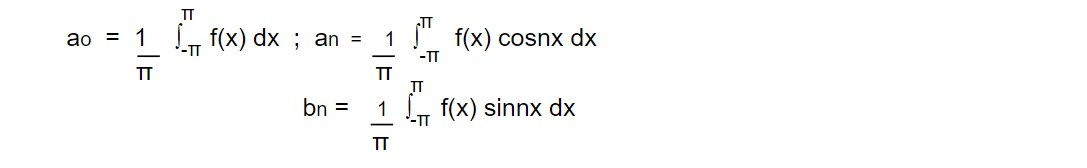The coefficients ao, an and bn are called Euler's coefficient.

Odd and even function :

A function f(x) is said to be odd function if

f(-x) = - f(x)

For example: f(x) =sin x, x^3

A function is said to be even function if

f(-x) = f(x)

For example: f(x) = cos x, x^2

A function f(x) is neither odd or even if

f(-x) ≠ - f(x) and f(-x) ≠ f(x)

For example: f(x) = e^-x

Fourier series for odd function

For an odd function f(x) such that f(-x) = -f(x), the Fourier series in interval [-π, π] is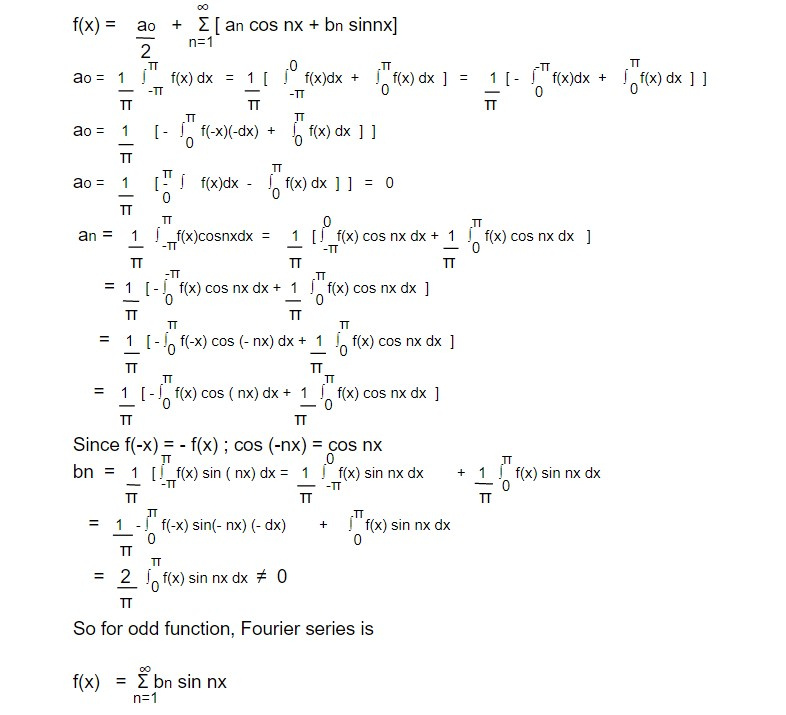Fourier Series for even function

Let f(x) be an even function i.e f(-x) = f(x). Then the Fourier series is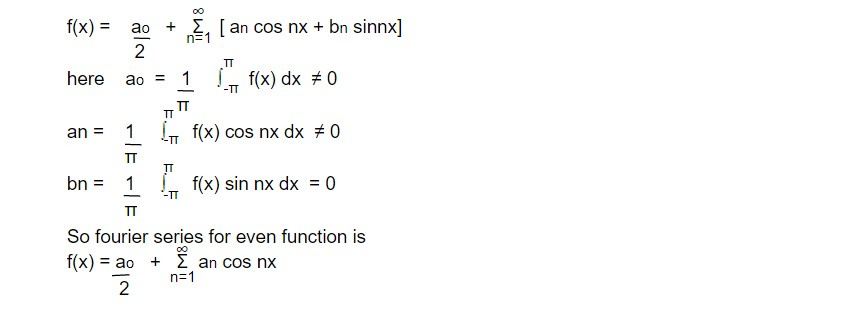Fourier series in interval [-l, l ]

The Fourier series of function f(x) in the interval [-π,π ] is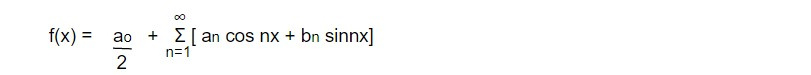where ao, an and bn are to be determined by Euler's formula. If we change interval from [-π,π ] to [-l, l ] then argument angle of cosine and sine changes from nx to nπx/l . So Fourier series for interval [-l, l ] is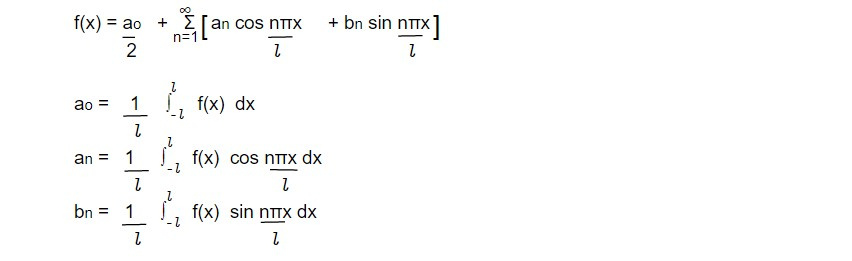Fourier series in complex form

If f(x) is periodic in interval [-l, l ], then fourier series isChanging cosine and sine function in complex form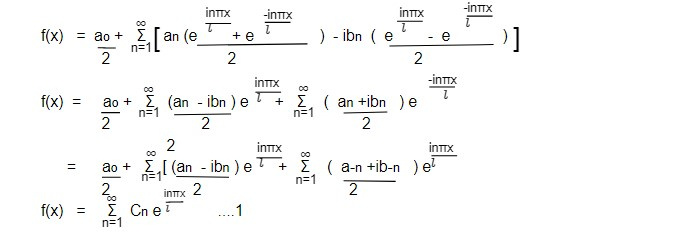Equation 1 is fourier series in complex form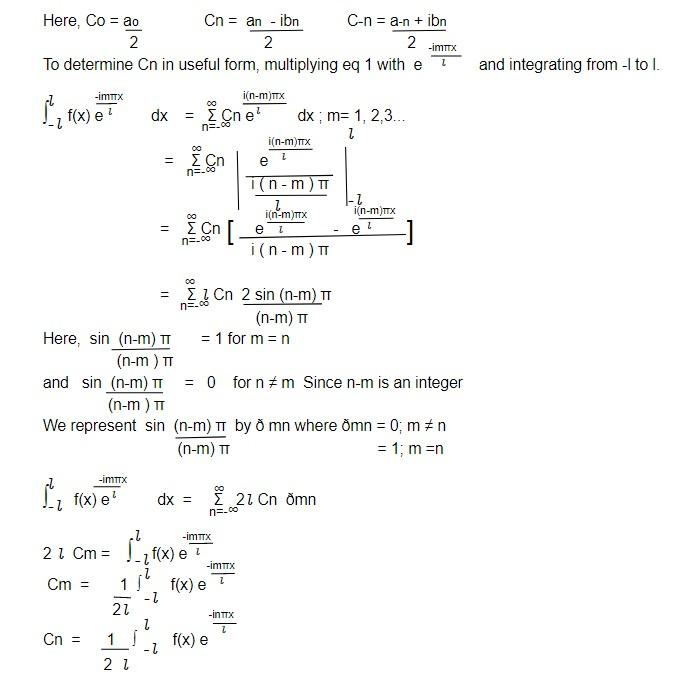This note is taken from Mathematical physics, MSC physics, Nepal.

This note is a part of the Physics Repository.